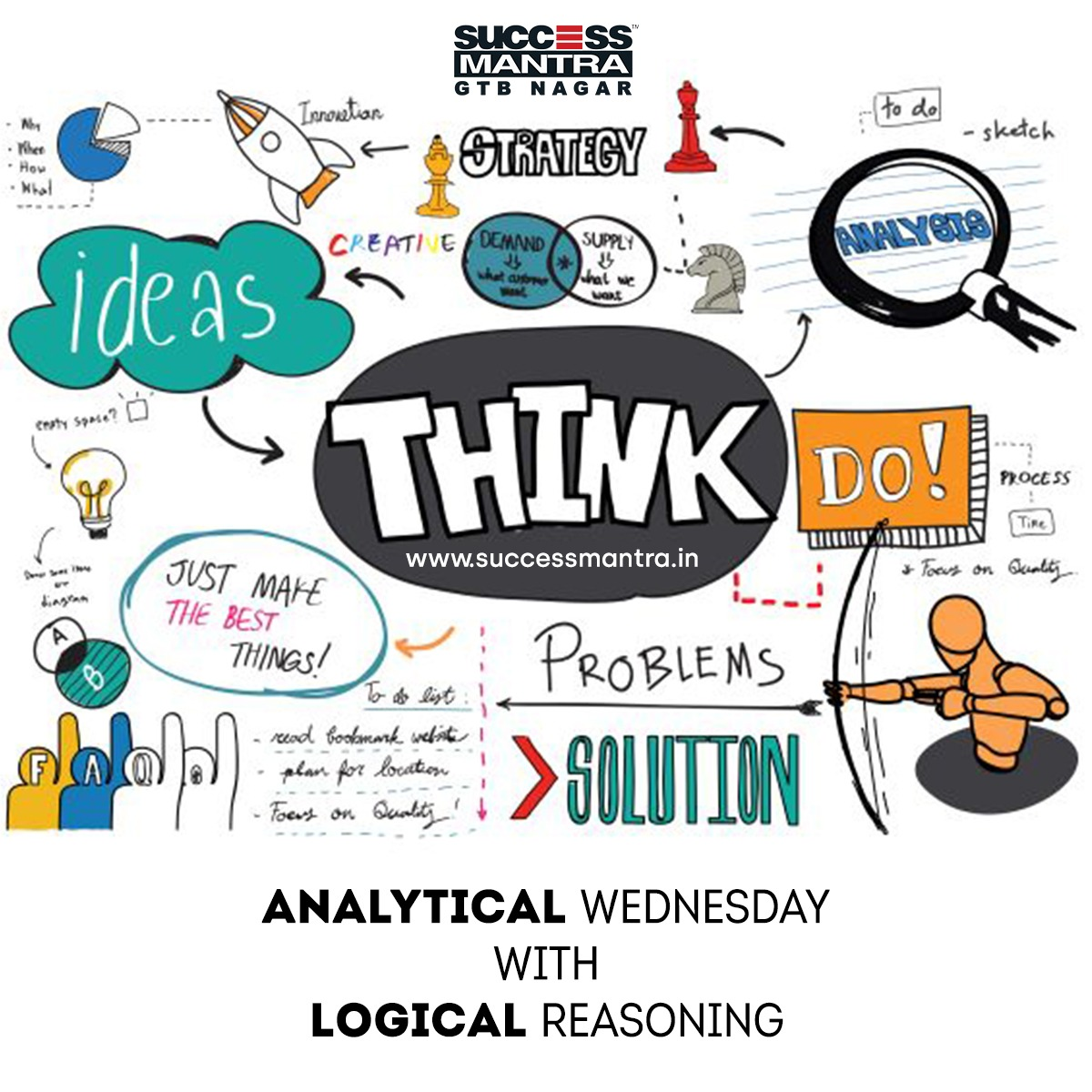Questions on Logical Reasoning SMLRQ035Directions: IN the following question, a group of numerals is given followed by four groups of symbol/letter combinations lettered (A), (B), (C), and (D). Numerals are to be coded as per the codes and conditions.
You have to find out which of the combinations (A), (B), (C) and (D) is correct and indicate your answer accordingly.

 Numerals 3 5 6 1 0 2 7 4 9 8 Letter/ Symbol @ D F # % R B ! & Q

Following conditions apply:
(i) If the number is a prime number the first digit is to be replaced by “@” and the last digit is to be replaced by “&”
(ii) If the number is a perfect square it is to be divided by two and the quotient is to be coded as per these conditions and the letter/ symbol code that would apply
(iii) If the first digit as well as the last digit is odd, both are to be coded as #
(iv) In case both (i) and (iii) are applicable condition number (i) will apply

1. Find out the combination for: 319602

a. #D&Q%#
b. @D&Q%&
c. @#&F%R
d. None of these

2. Find out the combination for 627

a. #RB
b. #R#
c. #BR
d. FRB

3. Find out the combination for 1936

a. #&@R
b. #FQ
c. &FQ
d. #&@F

4. Find out the combination for 773

a. #B#
b. [email protected]
c. @B&
d. #[email protected]

5. Find out the combination for 1000

a. D%%
b. #%%%
c. @%%&
d. None of these

1. Option c
None of the four conditions apply on the number so the digits will be coded to their letter/symbol equivalent. So 3 will become @, 1 = #, 9 = & and so on.

2. Option d
None of the four conditions apply on the number so the digits will be coded to their letter/symbol equivalent. So 6 will become F, 2 = R and 7 = B.

3. Option c
Since 1936 is a perfect square of 44 condition number (ii) will apply and the number to be coded will now be 1936/2 = 968. The correct combination is &FQ.

4. Option c
Since 773 is a prime number condition number (i) will apply.

5. Option b
None of the four conditions apply on the number so the digits will be coded to their letter/symbol equivalent. So 1 will become #, 0 = % and so on.